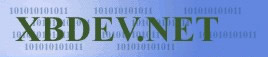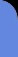Friday July 3, 2020
 home | about | contact | Donations

Basic Random Numbers

by bkenwright@xbdev.net

Have you ever wondered how srand() and rand() work in your stdlib.h C library's?  Well I'll show you how in just a sec, and you'll see just how easy it is...and of course how insecure it is.  But for most cases, rand() is perfect....

rand() in the stdlib.h C library uses the "Generator to linear congruenze" rule... and uses the formula:

 f(x) = (a*x + b) mod m

where m would be 231-2 for a 32 bit machine, a and b are constants.

 code: code.cpp //------------------------------------------------------------// //              Pseudo-random number generation               // //------------------------------------------------------------// static unsigned long int next = 1;   int rand(void) {   next = next * 1103515245 + 12345;   return (unsigned int)(next/65536) % 32768; }   void srand(unsigned int seed) {   next = seed; }   //------------------------------------------------------------// //                    Program Entry Point                     // //------------------------------------------------------------// void main() {       printf("Random Numbers:\n");       srand(1);         for(int i=0; i<10; i++)       {             int iRand = rand();             printf("%d \n", iRand);                   }// End for loop i   }// End of main()

 Output: Random Numbers: 16838 5758 10113 17515 31051 5627 23010 7419 16212 4086

As you can see where generating a pseudo random number, which is different each time in our loop.  Its interesting to note, that if you run the program a second time, you'll get an identical output as you'd expect...which is why you would usually seed your random number generator with some rand value to start of with, for example the time: "srand( (unsigned)time( NULL ) );" which would use <time.h> from the standard C library's.

Now to proove that the C code I've shown above is just as good as the one in the <stdlib.h> one...I'll repeat the experiment with the one included in the standard C library's...and see what result I get:

 code: code_stdlib.cpp #include    // need this for printf(..) #include   // for our srand() and rand()     //------------------------------------------------------------// //                    Program Entry Point                     // //------------------------------------------------------------// void main() {       printf("Random Numbers using the C algorithm:\n");       srand(1);         for(int i=0; i<10; i++)       {             int iRand = rand();             printf("%d \n", iRand);                   }// End for loop i   }// End of main()

 Output: Random Numbers using the C algorithm: 41 18467 6334 26500 19169 15724 11478 29358 26962 24464

Acckkk...its different than the value we have for our random numbers...why?... well its simply a difference of the values that your compiler has used... I'm using the Visual Studio compiler and, if I open up the rand.c file deep..deep within the installation I find this:

 int __cdecl rand (void) {          return(((holdrand = holdrand * 214013L + 2531011L) >> 16) & 0x7fff); }

See its not that much more different than ours.... but its named its seed value different and used a different set of constant variables.

Now a few things to note about the improved Visual C version..its using simple optimisation tricks...such as binary shift instead of multiplication :)  And if the modulous is a power of 2, then you can use a AND operation etc...which I urge you to look into, as its a great tip to know.  A possible further optimisation would be maybe to make it inline...or use _fastcall instead of __cdecl.

 Visitor: 9534626  { 209.237.238.175 } Copyright (c) 2002-2020 xbdev.net - All rights reserved. Designated tutorial and software are the property of their respective owners.